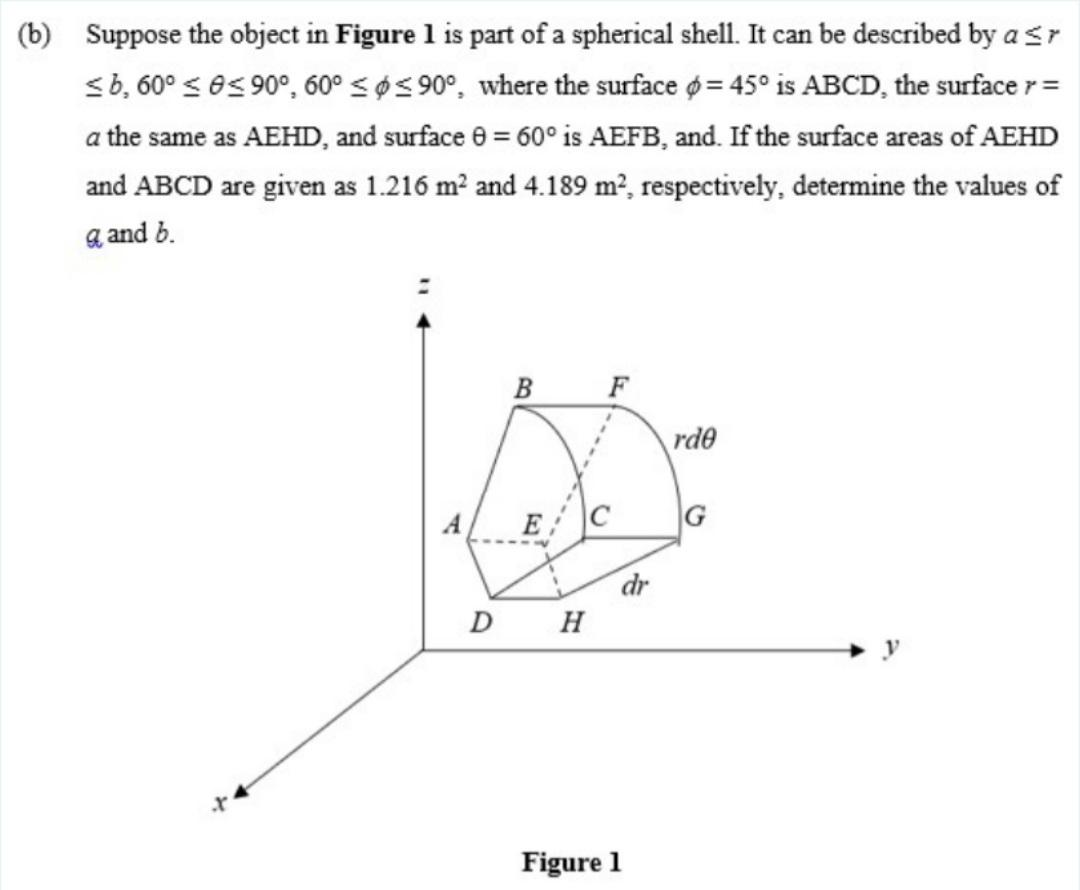# Question (b) Suppose the object in Figure 1 is part of a spherical shell. It can be described by asr 36,60º < 0< 90°, 60° < $900where the surface ø= 45° is ABCD, the surface r= a the same as AEHD, and surface 8 = 60° is AEFB, and. If the surface areas of AEHD and ABCD are given as 1.216 m2 and 4.189 m², respectively, determine the values of q and b. - B F rde А) E G dr DH Figure 1T3IZ26 The Asker · Electrical EngineeringTranscribed Image Text: (b) Suppose the object in Figure 1 is part of a spherical shell. It can be described by asr 36,60º < 0< 90°, 60° <$ 900where the surface ø= 45° is ABCD, the surface r= a the same as AEHD, and surface 8 = 60° is AEFB, and. If the surface areas of AEHD and ABCD are given as 1.216 m2 and 4.189 m², respectively, determine the values of q and b. - B F rde А) E G dr DH Figure 1
More
Transcribed Image Text: (b) Suppose the object in Figure 1 is part of a spherical shell. It can be described by asr 36,60º < 0< 90°, 60° < \$ 900where the surface ø= 45° is ABCD, the surface r= a the same as AEHD, and surface 8 = 60° is AEFB, and. If the surface areas of AEHD and ABCD are given as 1.216 m2 and 4.189 m², respectively, determine the values of q and b. - B F rde А) E G dr DH Figure 1